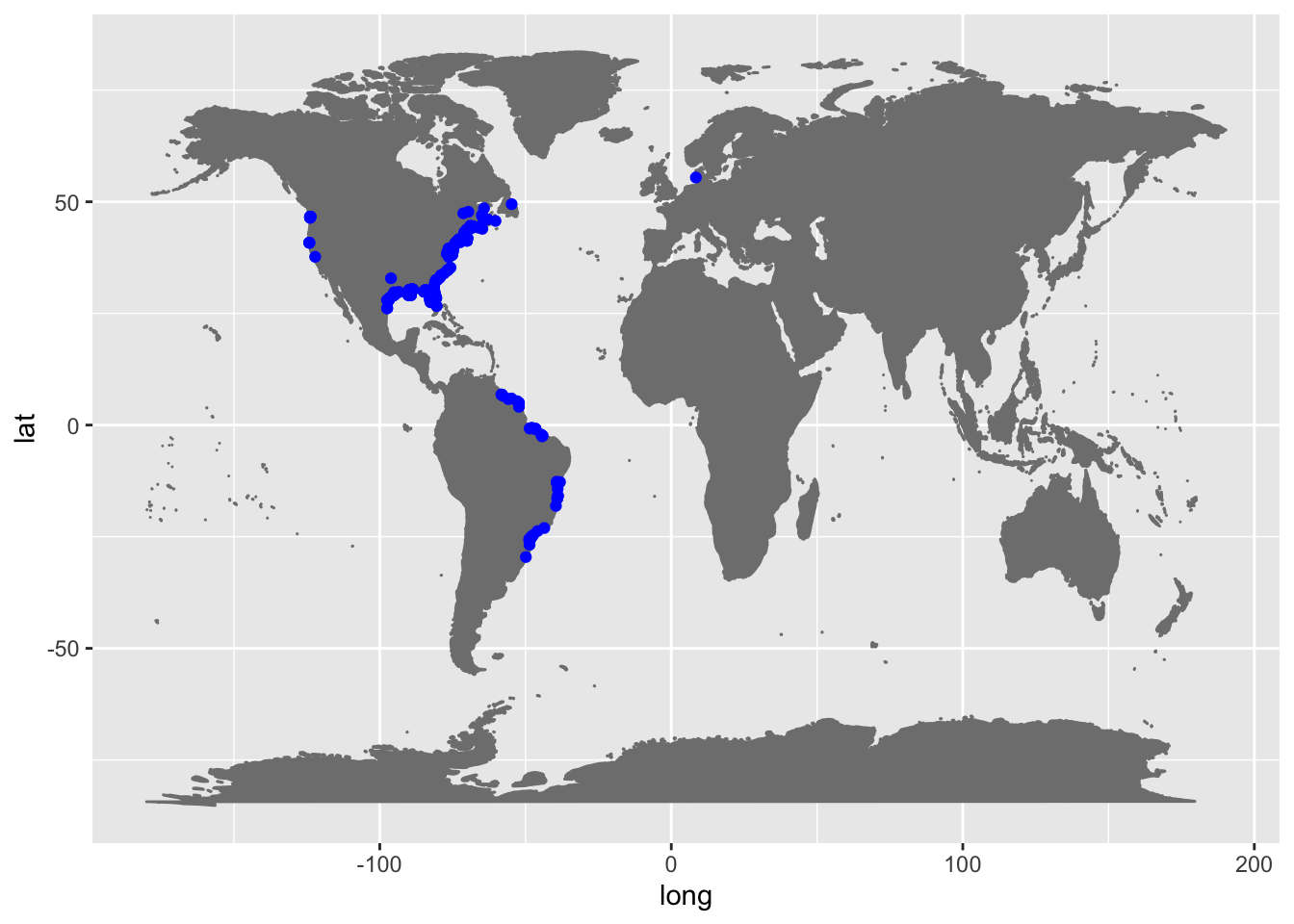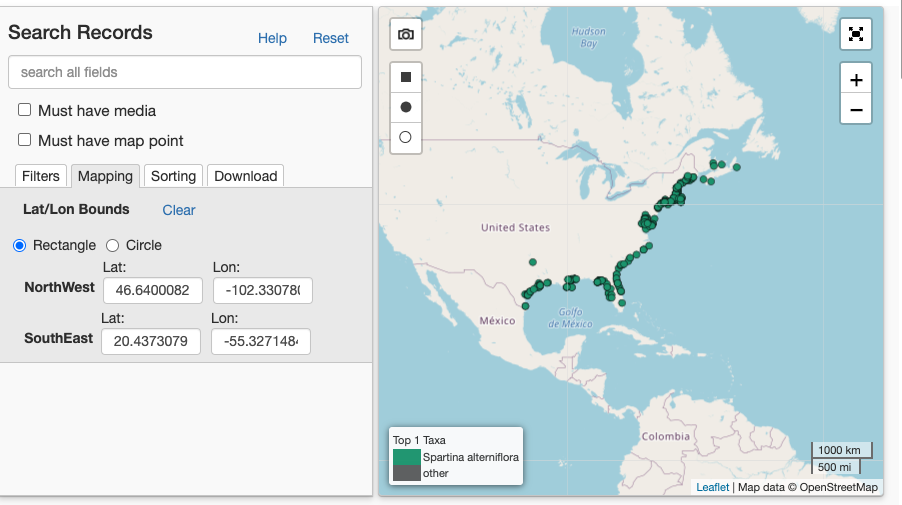This is a basic tutorial showing how to limit the extent of a download to a bounding box.

``````library(ridigbio)
library(ggplot2)``````

Here we download all the records for Spartina alterniflora, smooth cord grass, which is native to the eastern United States.

``iDigBio_SA <- idig_search_records(rq=list(scientificname="Spartina alterniflora"))``

### Plot the records

When you plot the occurrence records downloaded, you will notice points outside of the native range! Spartina alterniflora is very invasive in California and other parts of the world.

``````world <- borders(database = "world", colour = "gray50", fill = "gray50")
all_plot <- ggplot() +
world +
geom_point(iDigBio_SA,
mapping = aes(x = geopoint.lon, y = geopoint.lat),
col = "Blue")
all_plot``````## Limiting the extent

What if you want to only points for the species within the native extent?
Hint: Use the iDigBio portal to determine the bounding box for your region of interest.### Set the search criteria

Here we set a geo_bounding_box based on the iDigBio webportal - I limited the extent only to the native range.

``````rq_input <- list("scientificname"="Spartina alterniflora",
geopoint=list( type="geo_bounding_box",
top_left=list(lon = -102.33, lat = 46.64),
bottom_right=list(lon = -55.33, lat = 20.43) ) )``````
``iDigBio_SA_limited <- idig_search_records(rq=rq_input)``

### Plot the records

These records only include points within the desired extent!

``````USA <- borders(database = "usa", colour = "gray50", fill = "gray50")
limited_plot <- ggplot() +
USA +
geom_point(iDigBio_SA,
mapping = aes(x = geopoint.lon, y = geopoint.lat),
col = "Blue") +
coord_map(xlim = c(-60,-105), ylim = c(20, 50))

limited_plot``````